## Wednesday, November 24, 2021

### RLC Circuit: UNIZOR.COM - Physics4Teens - Waves - Electronic Oscillator

Notes to a video lecture on http://www.unizor.com

RLC Circuit

The previous lecture considered a simple circuit that contained a battery, a capacitor C and an inductor L, while ignoring an internal resistance of a circuit R.
This lecture continues the exploring the oscillating characteristics of this circuit, but, in addition, we will address more practical case of the existence of an electrical resistance R in the circuit.
These circuits are called RLC-circuits.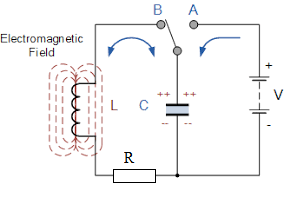As in the previous lecture, we put an AB-switch in the A position to charge the capacitor from a battery.
Then we switch to the B position, battery is no longer a factor, and capacitor is charged.

Initially, the voltage between the plates of a capacitor is at maximum, so the electric current is at maximum as well.
As the charge goes from one plate to another through an inductor and a resistor, the voltage and the electric current are diminishing.

Assume, the electric current in the circuit is changing with time t as I(t), the voltage drop between the plates of the capacitor is VC(t), the voltage drop on the inductor is VL(t) and the voltage drop on the resistor is VR(t).

By definition of the capacitance C of a capacitor,
C = Q(t)/VC(t) or
C·VC(t) = Q(t)
where Q(t) is the charge on its plates at time t.
We prefer to deal with the electric current I(t), which is defined as amount of moving charge per unit of time, which is mathematically expressed as a derivative of a charge by time,
I(t) = dQ(t)/dt

Differentiating the above equation between voltage and charge on a capacitor, we obtain the dependency between voltage and current on a capacitor:
dVC(t)/dt = I(t) or
V'C(t) = I(t)/C

Any current that goes along a wire creates a magnetic field around this wire. Since the electric current in our inductor is variable, the magnetic field that is generated by this current is variable.

According to the Faraday's Law, the variable magnetic field going through a wire loop generates electromotive force (EMF) equal to a rate of change of the magnetic field flux.
Therefore,
VL(t) = dΦ(t)/dt or
VL(t) = Φ'(t)

In turn, magnetic flux Φ(t) going through inductor, as a function of time t, is proportional to an electric current I(t) going through its wire
Φ(t) = L·I(t)
where L is a coefficient of proportionality that depends on physical properties of the inductor called inductance of the inductor.
From this we derive the following equation that describes the relationship between VL(t) and I(t):
VL(t) = L·I'(t)

According to the Ohm's Law, the voltage drop on the resistor is
VR(t) = R·I(t)

Finally, since the circuit contains no sources of electrical energy, sum of all voltage drops should be equal to zero:
VC(t) + VL(t) + VR(t) = 0

Now we have the following system of four equations with four unknown functions:
V'C(t) = I(t)/C
VL(t) = L·I'(t)
VR(t) = R·I(t)
VC(t) + VL(t) + VR(t) = 0

Differentiating the last equation by t and substituting voltages through their expressions in terms of electric current, we obtain
L·I"(t) + R·I'(t) + I(t)/C = 0

The last equation resembles the one that describes damped mechanical oscillations of an object on an ideal spring
m·x"(t) + c·x'(t) + k·x(t) = 0
where
x(t) is a displacement of an object on a spring from the neutral position,
m is an object's mass,
c is a damping coefficient,
k is a spring's elasticity
(see lecture UNIZOR.COM - "Physics 4 Teens" - "Waves" - "Mechanical Oscillations" - "Viscosity Damping 1")

As we described in the lecture "Viscosity Damping 1", the general solution to a homogeneous linear differential equation of the second order, like the one above, can be found by finding two functions that satisfy this equation x1(t) and x2(t), called partial solutions, and describing the general solution as a linear combination of these two functions
x(t) = A·x1(t) + B·x2(t)
The coefficients A and B depend on two initial conditions x(0) and x'(0).

In this case of electronic oscillation mathematics are exactly the same, which is quite remarkable.
Let's proceed with a solution along the same path as presented in the lecture on damped mechanical oscillations.

We will seek partial solutions based on function I(t) = eγ·t.
where γ - some (generally, complex) number. The reason for this is that, substituting this function with its first and second derivatives into the differential equation, we get
I'(t) = γ·eγ·t
I"(t) = γ²·eγ·t
and the differential equation is
γ²·L·eγ·t + γ·R·eγ·t + eγ·t/C = 0

Canceling eγ·t, which never equal to zero, we get a quadratic equation for γ
γ²·L + γ·R + (1/C) = 0
with any of its solutions (generally, complex), used in function eγ·t, delivering the partial solution of the original differential equation.

Since a quadratic equation has, generally speaking, two solutions among complex numbers γ1 and γ2, we have two solutions to our original differential equations:
I1(t)=eγ1·t and I2(t)=eγ2·t.

Moreover, since our equation is a homogeneous linear differential equation of the second order, all linear combinations of the above two solutions deliver a general solution that looks like
I(t) = A·eγ1·t + B·eγ2·t
where constants A and B are defined by initial conditions on a circuit.

Switching from theory to concrete results for our differential equation presented above, our first task is to solve the quadratic equation to find γ1 and γ2
The quadratic equation for γ is
γ²·L + γ·R + (1/C) = 0

This is exactly analogous to damped mechanical oscillations (see three "Viscosity Damping" lectures in the "Mechanical Oscillations" topics of this course), where the corresponding equation looks like
γ²·m + γ·c + k = 0
(m - mass, c - damping coefficient, k - elasticity of a spring).

Solutions to this equation that describes the (generally, complex) exponent of damped mechanical oscillations are
γ1,2 = −c/(2m)±√(c/(2m))²−k/m

Substituting inductance L of an electronic oscillator for mass m of mechanical one, resistance R of an electronic oscillator for damping coefficient c of mechanical one and inverse of capacitance 1/C of an electronic oscillator for elasticity k of mechanical one, we can use all the calculations of damped mechanical oscillations to obtain corresponding results for damped oscillations of electronic oscillator.

Solutions to our quadratic equation for γ, therefore, are:
γ1,2 = −R/(2L) ±
± √(R/(2L))²−1/(L·C)

Following the same logic as in "Viscosity Damping" lectures, we consider three cases:
Δ = (R/(2L))²−1/(L·C) > 0
Δ = (R/(2L))²−1/(L·C) = 0
Δ = (R/(2L))²−1/(L·C) < 0

Case 1: Δ > 0

Let
ω² = Δ = (R/(2L))²−1/(L·C), where ω is some positive number that is less than R/(2L)
Then both solutions to our quadratic equations are negative
γ1,2 = −R/(2L) ± ω < 0

General solution to our differential equation is
I(t) = A·e(−R/(2L)+ω)·t +
+ B·e(−R/(2L)−ω)·t

Both components of this general solution for I(t) with any coefficients A and B contain an exponential function with negative multiplier at time variable t.
That means, after some initial fluctuation, the electric current will be monotonously diminishing by absolute value, and there will be no oscillations.

This is an overdamped circuit, analogous to overdamping of mechanical oscillations within a viscous environment with high viscosity.
High viscosity of the environment for mechanical oscillations is equivalent to high resistance of the electrical circuit.

The graph of electrical current I(t) as a function of time t would look similar to this one: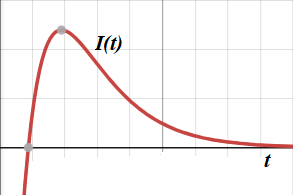Case 2: Δ = 0

In this case
γ1,2 = −R/(2L) ± 0 = −R/(2L)
Unfortunately, in this case our quadratic equation has only one solution, we will call it γ1.

But, to come up with a general solution to a homogeneous linear differential equation of the second order we need two linearly independent partial solution, like in Case 1 above.

To look for a second solution, we will expand our search and, following the same logic as in "Viscosity Damping 2" lecture of "Mechanical Oscillations" topic, we will try to find a solution to our differential equation in the form
I(t) = t·eγ·t

There is one particular value of variable γ that makes this function a solution to our differential equation. Just substituting this function together with its first and second derivatives in the differential equation renders the value
γ2 = −R/(2L)
as it was derived in the lecture "Viscosity Damping 2".

Therefore, we have now two linearly independent partial solutions to our differential equation:
I1(t) = eγ1·t = e−(R/(2L))·t
I2(t) = t·eγ2·t = t·e−(R/(2L))·t
General solution to our differential equation is
I(t) = A·e−(R/(2L))·t +
+ B·t·e−(R/(2L))·t

Since all exponents are negative number multiplied by time, the asymptotic behavior of this function is similar to the one presented in Case 1 - after some initial fluctuation, the electric current will be monotonously diminishing by absolute value, and there will be no oscillations.

The graph of electrical current I(t) as a function of time t would look similar to the one of Case 1 above.

Case 3: Δ < 0

Let
ω² = −Δ = −(R/(2L))²+1/(L·C), where ω is some positive number.
Then both solutions to our quadratic equations are complex numbers
γ1,2 = −R/(2L) ± ω·i

One particular solution to our differential equation is a function with complex values
I1(t) = eγ1·t = e(−R/(2L)+ω·i)·t
and another is
I2(t) = eγ2·t = e(−R/(2L)−ω·i)·t

If a function with complex values, like Z(t)=X(t)+i·Y(t), where X(t) and Y(t) are real values functions and i²=−1, satisfies a linear differential equation, its real and imaginary components must satisfy this equation too, because, if a+b·i=0 then a=0 and b=0 too.

To represent functions I1,2(t) above in a canonical form for complex numbers, we will use the Euler's formula
ei·x = cos(x) + i·sin(x)

Therefore,
I1(t) = e(−R/(2L)·t·
·
[cos(ω·t) + i·sin(ω·t)]
I2(t) = e(−R/(2L)·t·
·
[cos(ω·t) − i·sin(ω·t)]

Since real and imaginary parts of a solution to a linear differential equation must be solutions in their own right, function I1(t) gives two linearly independent real valued partial solutions
I1a(t) = e(−R/(2L)·t·cos(ω·t)
and
I1b(t) = e(−R/(2L)·t·sin(ω·t)

Similarly, function I2(t) gives two linearly independent real valued partial solutions
I2a(t) = e(−R/(2L)·t·cos(ω·t)
and
I2b(t) = −e(−R/(2L)·t·sin(ω·t)

Functions I2a(t) and I2b(t) are linearly dependent on I1a(t) and I1b(t) and are not needed to construct a general solution to our differential equation. From partial solutions I1a(t) and I1b(t) we can construct a general solution to our differential equation
I(t) = e(−R/(2L)·t·
·
[A·cos(ω·t) + B·sin(ω·t)]

The electric current of this kind gives true oscillations, albeit damped ones with an amplitude exponentially diminishing because of the presence of a resistor in a circuit.
Its graph looks like this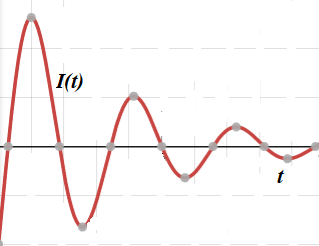## Saturday, November 20, 2021

### Electronic Oscillator: UNIZOR.COM - Physics4Teens - Waves - Oscillations...

Notes to a video lecture on http://www.unizor.com

Electronic Oscillator

From the "Electromagnetism" part of this course we know that variable (sinusoidal) oscillations of electric current in the wire frame can be induced by rotating this frame in the magnetic field.
Using the terminology of the mechanical oscillations, the oscillations of the electric current in such a case should be called forced.

There are, however, circuits where oscillations of electric current are free from any external forces.
Let's consider the following simple circuit that contains a battery, a capacitor C and an inductor L, ignoring for now an electrical resistance of a circuit.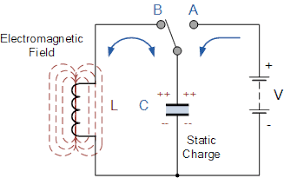The above is the LC-circuit because it consists of an inductor and a capacitor.

When an AB-switch is in the A position, the capacitor will be charged from a battery.
Now we switch to the B position, battery is no longer a factor, and capacitor is charged.

Initially, the voltage between the plates of a capacitor is at maximum, so the electric current is at maximum as well.
As the charge goes from one plate to another through an inductor, the voltage and the electric current are diminishing sinusoidally from some maximum at time t=0 to zero at t=T/4, where T is a period.

Assume (and the supporting calculations of the validity of this assumption follow), the electric current is diminishing with time t as
I(t) = I0·cos(ωt)
where
I0 is the maximum value of a current
ω is angular speed
T = 2π/ω is a period

The speed of change of the current is the first derivative of this function
dI/dt = −I0·ω·sin(ωt)
This speed of change also changes with time sinusoidally, but its maximum at t=T/4 coincides with minimum of the current itself.

As we know, the change in the current in the inductor causes the change of the magnetic field around it, which, in turn, causes the appearance of a self-inducted electromotive force. You can refer to "Self-induction" chapter of the "Electromagnetism" part of this course for details.

The electromotive force is stronger, when the speed of change of the magnetic field is stronger, and it forces the electric current to continue its existence even after one plate of the capacitor lost its charge to another plate.

The maintained by the magnetic field current will make the capacitor to be charged again, but in opposite direction (the plate, that used to positive, now is negative and vice versa).

As the charge accumulates on the capacitor, it slows down and stops the flow of the current induced by a changing magnetic field. When the current is stopped, the situation resembles the one at time t=0, but with reversed charges on the capacitor's plates. So, the current starts flowing again, but in opposite direction.

The internal resistance R of a circuit will result in some lost energy and, therefore, the maximum charge on the plates of a capacitor will diminish with time. This exactly resembles damped mechanical oscillations.

Let's get into some theory of this LC-circuit without taking into consideration the internal resistance of a circuit.
Assume that the current in a circuit is I(t), the voltage drop between the plates of the capacitor is VC(t) and the voltage drop on the inductor is VL(t).

By definition of the capacitance C of a capacitor,
C = Q(t)/VC(t) or
C·VC(t) = Q(t)
where Q(t) is the charge on its plates at time t.
We prefer to deal with the electric current I(t), which is defined as amount of moving charge per unit of time, which is mathematically expressed as a derivative of charge by time,
I(t) = dQ(t)/dt

Differentiating the above equation between voltage and charge on a capacitor, we obtain the dependency between voltage and current on a capacitor:
dVC(t)/dt = I(t) or
V'C(t) = I(t)/C

Any current that goes along a wire creates a magnetic field around this wire. Since the electric current in our inductor is variable, the magnetic field that is generated by this current is variable.

According to the Faraday's Law, the variable magnetic field going through a wire loop generates EMF equal to a rate of change of the magnetic field flux.
Therefore,
VL(t) = dΦ(t)/dt or
VL(t) = Φ'(t)

In turn, magnetic flux Φ(t) going through inductor, as a function of time t, is proportional to an electric current I(t) going through its wire
Φ(t) = L·I(t)
where L is a coefficient of proportionality that depends on physical properties of the inductor called inductance of the inductor.
From this we derive the following equation for VL(t):
VL(t) = L·I'(t)

Finally, since the circuit contains no sources of electrical energy, sum of all voltage drops should be equal to zero:
VC(t) + VL(t) = 0

Now we have the following system of three differential equations with three functions:
V'C(t) = I(t)/C
VL(t) = L·I'(t)
VC(t) + VL(t) = 0

Differentiating the third equation by t and substituting voltages through their expressions in terms of electric current, we obtain
I(t)/C + L·I"(t) = 0 or
I"(t) + I(t)/(L·C) = 0

The last equation resembles the one that describes harmonic mechanical oscillations of an object on an ideal spring
x"(t) + (k/m)·x(t) = 0
where
x(t) is a displacement of an object on a spring from the neutral position,
k is a spring's elasticity and
m is an object's mass.

The general solution to the above equation, as we have shown, when considered the periodic mechanical oscillations, is
x(t) = C1·cos(ω·t) + C2·sin(ω·t)
where
ω² = k/m
C1 is initial displacement of the object from the neutral position of equilibrium
C2 is defined by the initial velocity of an object and is zero, if there is no initial velocity.

Analogously, the general solution of our differential equation for the electric current in an LC-circuit is
I(t) = C1·cos(ω·t) + C2·sin(ω·t)
where
ω² = 1/(L·C)
C1 and C2 are defined by initial conditions.

The period of these oscillations of electric current in the LC-circuit is
T = 2π/ω = 2π√L·C
This is known as the Thomson's formula.

## Sunday, November 14, 2021

### Problems 4: UNIZOR.COM - Physics4Teens - Waves - Mechanical Oscillations

Notes to a video lecture on http://www.unizor.com

Problems 4

Problem A

As presented in the lecture "Forced Oscillation 1", the general solution to a differential equation that describes forced oscillation looks like
x(t)=F0·cos(ω·t)/[m(ω0²−ω²)] +
+ D·cos(ω0·t−φ)

where
F0 is an amplitude of a periodic external force;
ω - angular speed of a periodic external force;
m - mass of an object on a spring;
k - elasticity of a spring;
ω0=√k/m is an inherent natural angular speed of oscillation of this particular object on this particular spring;
t is time;
D and φ parameters are determined by initial conditions for x(0) and x'(0).

Consider forced oscillations used as an example in that lecture.
A picture below represents forced oscillations of a system with its own natural angular speed ω0=2(rad/sec) with periodic force applied to it that has its angular speed ω=5(rad/sec).
Initial displacement and speed are at zero.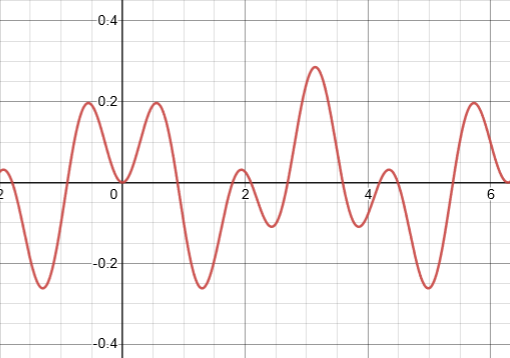Are the forced oscillations periodic?
If yes, what is the period of oscillations?

Solution

The result of a periodic force acting on periodic movement of the system is a periodic movement of this system but with a longer period.
If a period of a force is T1 and the own natural period of a system T2, then smallest number T that is a multiple of both T1 and T2 (if it exists!) will be a period of system with periodic external force acting on it.

Such a period will exist, if T1/T2 is a rational number.
Indeed, if T1/T2=m/n, then T=T1·n=T2·m will be divisible by T1, getting integer n as a result, and by T2, getting integer m as a result.

The period of an external force with angular speed ω=5(rad/sec) is
T1 = 2π/5(sec)
The natural period of a system with its own natural angular speed ωh=2(rad/sec) is
T2 = 2π/2 = π(sec)
In this case T=2π(sec) would be a smallest time interval that contains integer number of both periods T1 (T/T1=2) and T2 (T/T2=5).

The system will be in a periodic motion with a period T=2π(sec).

Problem B

Let's solve a problem that combines free oscillations, viscosity and external periodic force acting on an oscillating object.
Making all the reasonable assumptions, derive the equation of motion of such oscillations.

Solution

Let's assume that oscillations and all the forces are acting along the X-axis, so we have to derive a position of an object relative to the equilibrium point, which is at the origin of X-coordinate, as a function of time t.

If the position of an object relative to an equilibrium point is x(t), we assume the following:
(a) a spring acts on it, according to the Hooke's Law, with a force Fs(t)=−κ·x(t), where κ is a spring elasticity
(b) viscosity force is proportional to the speed x'(t) of an object and equals to Fv(t)=−c·x'(t), where c is viscosity damping coefficient
(wherever necessary, we will assume that viscosity c is sufficiently small in order to observe some oscillations)
(c) an external force is periodic and equals to Fx(t)=F0·cos(ω·t), where F0 is a constant amplitude and ω represents an angular speed of oscillations of an external force.

Now, according to the Newton's Second Law, the combination of all these forces should be equal to mass m of an oscillating object times its acceleration x"(t):
m·x"(t) = −κ·x(t) − c·x'(t) +
+ F0·cos(ω·t)

It can be transformed into more traditional format
x"(t)+(c/m)·x'(t)+(κ/m)·x(t) =
= (F0/m)·cos(ω·t)

This differential equation is not homogeneous because the right side contains some function that is not linearly dependent on x(t).
If two solutions of the above non-homogeneous differential equation are known, their difference is, obviously, a solution to a corresponding homogeneous equation
xh"(t) + (c/m)·xh'(t) +
+ (κ/m)·xh(t) = 0

Therefore, to find all solutions to a non-homogeneous differential equation, it is sufficient to find one particular solution xp(t) and add to it a general solution to a corresponding homogeneous equation xh(t).

Let's start by finding a general solution to the corresponding homogeneous differential equation
xh"(t) + (c/m)·xh'(t) +
+ (κ/m)·xh(t) = 0

It's a linear differential equation of the second order, so its general solution is any linear combination of any two linearly independent particular solutions.

Let's look for solutions to this differential equation among functions that look like this:
xh(t) = eγt
to find a pair of γ's (may be complex) that result in eγt to be a solution of our homogeneous differential equation.

Using this approach, we obtain
xh'(t) = γ·eγt
xh"(t) = γ²·eγt
Substituting this into our homogeneous equation:
γ²·eγt+(c/m)·γ·eγt+(κ/m)·eγt=0

Since eγt is not zero, we have a quadratic equation for γ
γ² + (c/m)·γ + (κ/m) = 0

Its discriminant is
Δ = (c/m)²−4κ/m
For sufficiently small (by assumption) viscosity c this discriminant will be negative.
So, our quadratic equation for γ will have two complex solutions:
γ1,2 = −c/(2m)±√(c/(2m))²−κ/m

Let
ωh² = −(c/(2m))² + k/m
Then
γ1,2 = −c/(2m)±√−ωh² =
= −c/(2m) ± ωh·i

where i is an imaginary unit, i²=−1

From this, the solution to our homogeneous differential equation, that does not take into account external periodic force, is
xh1,2(t) = eγ1,2·t =
= e
[−c/(2m)±ωh·i]·t =
= e−c·t/(2m)·e±ωh·i·t

The first member of this product e−c·t/(2m) is an exponentially diminishing amplitude of oscillation (because of viscosity).
The second one, according to Euler's formula, is
e±ωh·i·t = cos(ωh·t) ± i·sin(ωh·t)

If function z(t)=p(t)+i·q(t) with complex values satisfies some linear differential equation with real coefficients, its real and imaginary components must satisfy this equation independently.

Therefore,
xh1(t) = e−c·t/(2m)·cos(ωh·t)
and
xh2(t) = e−c·t/(2m)·sin(ωh·t)
are two independent solutions and the general solution to our linear homogeneous equation of the second order is any linear combination of these two solutions:
xh(t) = e−c·t/(2m)·
·
[A·cos(ωh·t)+B·sin(ωh·t)]
where A and B are any real numbers.

Using simple trigonometric identity
cos(α−β) =
= cos(α)·cos(β) + sin(α)·sin(β)

the above expression can be transformed into
xh(t) = R·e−c·t/(2m)·cos(ωh·t−φ)
where R = √A²+B² and
cos(φ)=A/R, sin(φ)=B/R.
Parameters R and φ are defined by initial conditions - initial displacement of an object from the equilibrium xh(0) and initial speed xh'(0).

Let's check that the above function is indeed a solution to our homogeneous differential equation
xh"(t) + (c/m)·xh'(t) +
+ (κ/m)·xh(t) = 0

Calculate all the components:
xh(t) = R·e−c·t/(2m)·cos(ωh·t−φ)

xh'(t) =
= −
[R·c/(2m)]·e−c·t/(2m)·
·cos(ωh·t−φ) −
[R·ωh]·e−c·t/(2m)·
·sin(ωh·t−φ)

xh"(t) =
=
[R·(c/(2m))²]·e−c·t/(2m)·
·cos(ωh·t−φ) +
+
[R·ωh·c/(2m)]·e−c·t/(2m)·
·sin(ωh·t−φ) +
+
[R·ωh·c/(2m)]·e−c·t/(2m)·
·sin(ωh·t−φ) −
[R·ωh²]·e−c·t/(2m)·
· cos(ωh·t−φ) =
= R·e−c·t/(2m)·
·
{[(c/(2m))²−ωh²]·cos(ωh·t−φ) +
+
[(c/m)·ωh]·sin(ωh·t−φ)}

(c/m)·xh'(t) =
= −
[R·c²/(2m²)]·e−c·t/(2m)·
·cos(ωh·t−φ) −
[R·ωh·c/m]·e−c·t/(2m)·
·sin(ωh·t−φ)

Since
κ/m = ωh² + (c/(2m))²
we get the following:
(κ/m)·xh(t) =
=
[ωh²+(c/(2m))²]·xh(t) =
=
[ωh²+(c/(2m))²]·R·e−c·t/(2m)·
·cos(ωh·t−φ)

Therefore,
xh"(t) + (c/m)·xh'(t) +
+ (κ/m)·xh(t) =
= R·e−c·t/(2m)·cos(ωh·t−φ)·X +
+ R·e−c·t/(2m)·sin(ωh·t−φ)·Y

where
X = (c/(2m))² − ωh² −
− c²/(2m²) + ωh²+(c/(2m))² =
= c²/(4m²) − ωh² −
− c²/(2m²) + ωh² + c²/(4m²) = 0

Y = (c/m)·ωh − ωh·c/m = 0

That proves that the general solution to our homogeneous differential equation of the second order is the function
xh(t) = R·e−c·t/(2m)·cos(ωh·t−φ)
where R is an amplitude and φ is a phase shift of oscillations, both determined by initial conditions xh(0) and xh'(0).

Now, to find all solutions to our original non-homogeneous differential equation
x"(t)+(c/m)·x'(t)+(κ/m)·x(t) =
= (F0/m)·cos(ω·t)

it is sufficient to find one particular solution xp(t) and add to it a general solution to a corresponding homogeneous equation xh(t) that we have found above.

Considering the trigonometric character of the right side of our original equation, let's try to find a particular solution in a form
xp(t) = A·cos(ω·t) + B·sin(ω·t)
where A and B are some constants.

Then
x'p(t) =
= −A·ω·sin(ω·t)+B·ω·cos(ω·t)

x"p(t) =
= −A·ω²·cos(ω·t)−B·ω²·sin(ω·t)

Substitute these functions into our original differential equation, getting −A·ω²·cos(ω·t)−B·ω²·sin(ω·t)−
−(c/m)·A·ω·sin(ω·t)+
+(c/m)·B·ω·cos(ω·t)+
+(κ/m)·A·cos(ω·t)+
+(κ/m)·B·sin(ω·t) =
= (F0/m)·cos(ω·t)

In order for this to be true for any time moment t, the corresponding coefficients at cos(.) and sin(.) functions on the left and right side of an equation must be equal.

The coefficient at cos(.) on the left side is supposed to be equal to (F0/m):
−A·ω²+(c/m)·B·ω+(κ/m)·A =
= (F0/m)

There is no sin(.) on the right side, so the coefficient at sin(.) on the left side is supposed to be equal to zero:
−B·ω²−(c/m)·A·ω+(κ/m)·B = 0

So, we have two linear equations with two variables A and B, which can easily be solved
−A·ω²+(c/m)·B·ω+(κ/m)·A =
= (F0/m)

−B·ω²−(c/m)·A·ω+(κ/m)·B =
= 0

Transform into canonical form
((k/m)−ω²)·A+(c/m)·ω·B =
= (F0/m)

−(c/m)·ω·A+((κ/m)−ω²)·B =
= 0

Solutions are:
A = (F0/m)·(k/m−ω²)/det
B = (F0/m)·(c/m)/det
where determinant
det = ((k/m)−ω²)² + (c/m)²·ω²

Now the general solution x(t) to our original non-homogeneous differential equation is a combination of the above partial solution
xp(t)=A·cos(ω·t)+B·sin(ω·t)
(where constants A and B are defined above)
and the general solution to a corresponding homogeneous solution
xh(t) = R·e−c·t/(2m)·cos(ωh·t−φ)

That is,
x(t) = xp(t) + xh(t) =
= A·cos(ω·t) + B·sin(ω·t) +
+ R·e−c·t/(2m)·cos(ωh·t−φ)

What's interesting about this analytical expression of such a complex oscillations is the fact that a component related to damped oscillations with the system's natural angular speed ωh is gradually decreasing in amplitude as time passes, because viscosity exponentially diminishes its amplitude R·e−c·t/(2m) to zero.
That leaves the oscillations with major angular speed that depends only on the driving angular speed ω of an external force.

The following graph renders the gradual transformation of damped oscillations under an external periodic force into oscillations mostly dependent on the angular speed of that external force and less and less dependent on the natural properties of a spring and environment.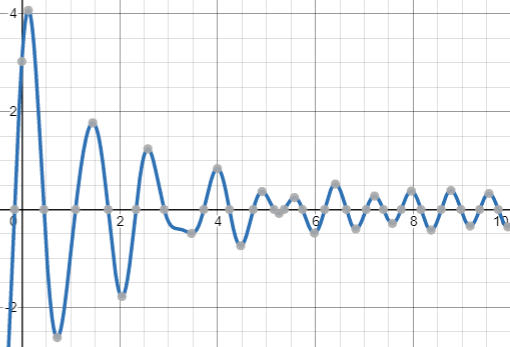The parameters chosen for this graph are:
A = 0.2
B = 0.3
ω =8
R = 4
c = 1
m = 1
ωh=5
φ = π/4

## Saturday, November 6, 2021

### Problem 3: UNIZOR.COM - Physics4Teens - Waves - Mechanical Oscillations

Notes to a video lecture on http://www.unizor.com

Problems 3

Problem A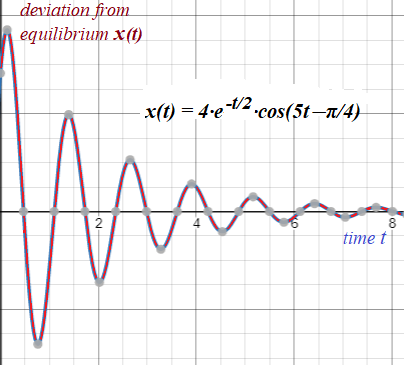An object, oscillating in viscous environment, moves according to the following equation of motion
x(t) = 4·e-t/2·cos(5t−π/4)
where t is time and x(t) is deviation from the equilibrium.

Determine the moments of time {ti} = {t0, t1, t2, ...}, when the object returns to the equilibrium.

Solution

Since we are talking about damping oscillations with diminishing displacements from the equilibrium, the motion is not exactly periodic, but we use the term period in a sense that an object returns to the equilibrium point again and again, albeit deviating from it less and less.

In this context period means the interval of time between consecutive crests or consecutive troughs of the trajectory, or between moments in time, when an object returns to the equilibrium position after reaching its local maximum deviation in both directions from the equilibrium.

Calculations below show that this interval does not change with time and remains constant, while the amplitude of oscillations around the equilibrium gets smaller with time.

To find the times when the object returns to the equilibrium, we have to find points, where x(t)=0.
Since coefficient in front of cos(...) never equals to zero, the solutions of an equation x(t)=0 are those moments in time, when cos(5t−π/4)=0.

Solving the equation:
cos(5t−π/4) = 0
5t−π/4 = π/2+N·π
5t = 3π/4+N·π
t = 3π/20+N·π/5
t = (3+4N)·π/20
where N ≥ 0 - an integer.

Interval between any two consecutive time moments of return to the equilibrium equals to π/5 (constant).
This makes a period of oscillation to be 2π/5 to allow an object to move from the equilibrium to one direction, return to the equilibrium, move to another direction and return again.

Substituting N=0,1,2..., we will get values {t0=3π/20, t1=7π/20, t2=11π/20, ...}
The first few members of this sequence are
t0 ≅ 0.471
t1 ≅ 1.100
t2 ≅ 1.728

{ti} = (3+4N)·π/20
where N ≥ 0 - an integer.

Problem B

Consider the same object and environment as in Problem A.
Determine the moments of time {τi} = {τ0, τ1, τ2, ...}, when the absolute value of object's deviation from the equilibrium reaches its local maximum.

Solution

Local extremes (maximum and minimum) of function x(t) are characterized by the fact that the derivative x'(t) at these points equals to zero.
x'(t) = −2·e-t/2·cos(5t−π/4) −
− 20·e-t/2·sin(5t−π/4) = 0

From this follows
cos(5t−π/4)+10·sin(5t−π/4)=0
tan(5t−π/4) = −1/10
5t−π/4 = arctan(−1/10) + N·π
5t = arctan(−1/10) +
+ (4N+1)·π/4

t = 0.2·arctan(−1/10) +
+ (4N+1)·π/20

where N ≥ 0 - an integer.

Since
arctan(−1/10) ≅ −0.0996686525,
the first few members (N=0,1,2) of this sequence are
τ0 ≅ 0.137 (local max)
τ1 ≅ 0.765 (local min)
τ2 ≅ 1.394 (local max)

{τi} = 0.2·arctan(−1/10) +
+ (4N+1)·π/20

where N ≥ 0 - an integer.

Problem C

Determine the values of object's deviations from the equilibrium {A0, A1, A2, ...}, when they are at local maximums by absolute value.

Solution

Ai = x(τi)
Therefore, the first few members of this sequence are
A0 ≅ 3.716 (local max)
A1 ≅ −2.714 (local min)
A2 ≅ 1.983 (local max)

{Ai} = {4·eτi·cos(5τi−π/4)}
where values {τi} were determined in Problem B.

## Thursday, November 4, 2021

### Problems 2: UNIZOR.COM - Physics4Teens - Waves - Mechanical Oscillations

Notes to a video lecture on http://www.unizor.com

Problems 2

Problem A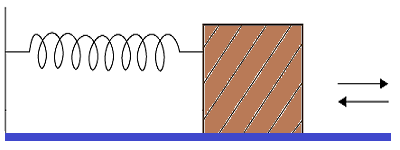An object of mass m=0.3 kg sits on a table and is attached to a wall by a horizontal spring with elasticity κ=2 N/m.
This object can slide left and right on a surface of a table with the static and dynamic friction coefficient μ=0.1.
Initially, the spring is in equilibrium position, not stretched, not squeezed.
What is the maximum distance λ we can stretch a spring such that the object would remain in that position on a stretched spring and not moved back towards equilibrium?
Assume that gravity acceleration is g=9.8 m/sec².

Solution

The necessary condition for an object to remain in place with a stretched spring is that the force of a spring that pulls the object back to equilibrium Fs is not sufficient to overcome the static friction force Ff.
Fs = κ·d = 2·d (N)
Ff = μ·m·g = 0.1·0.3·9.8 (N)
The necessary condition for an object to remain in place on a stretched spring is
Fs ≤ Ff
That is,
2(N/m)·λ(m) ≤ 0.1·0.3·9.8 (N)
from which follows
λ ≤ 0.147 (m)

The maximum distance to stretch a spring with an object not being pulled back (critical distance) is
λ = 0.147m = 147mm

Problem B

Consider the same set-up as in the Problem A.
Our task now is to stretch a spring beyond the critical distance λ, so that the object will be pulled back by a spring, but it should reach the equilibrium point and stop there with no further movements.
What is the distance d to stretch a spring to achieve this result?

Solution

Let's approach this problem from the energy standpoint.
As we know, the potential energy of a spring stretched by a distance d is
U(d) = κ·d²/2
(see "Energy of Oscillation" lecture in this chapter of a course)
Using the data from Problem A,
U(d) = 2·d²/2
This energy should be fully used to overcome the constant friction force Ff on a distance d.
Ff = μ·m·g = 0.294 (N)
On a distance d the force Ff performs work
W(d) = Ff·d = 0.294·d
which should be equal to initial potential energy U(d).
Therefore,
W(d) = U(d)
0.294·d = 2·d²/2
d = 0.294 (m)

In order to initiate a movement of an object back to the equilibrium point such that it will stop at that point, we have to stretch the spring by
0.294m = 294mm

Incidentally, this is a double critical distance, which could've been predicted since the initial point and a point of stop must be symmetrical relative to a critical point, as was proved in the "Energy of Oscillation" lecture.

## Wednesday, November 3, 2021

### Field Waves: UNIZOR.COM - Physics4Teens - Waves

Notes to a video lecture on http://www.unizor.com

Field Waves

So far, our understanding of waves was very much related to a concept of some material object to oscillate, that is some material particles going back and forth, up and down, left and right or, in short, making some repetitive movements.

But Physics deals not only with material in a usual sense objects. A very important part of what physicists deal with is fields.

Let's spend a few minutes to talk about a nature of the concept field.

In Mathematics it's customary to analyze the properties of some mathematical objects (like N-dimensional vector space) without really having any real life object that corresponds exactly to this mathematical concept.

Mathematicians care only about properties, regardless of the objects that possess these properties. Moreover, there might be completely different mathematical objects that possess exactly the same properties, and the beauty of Mathematics allow us not to think about objects that possess these properties, but to concentrate only on analysis of the properties themselves.

With properly analyzed properties, mathematicians can apply the results to any mathematical object that possess these properties.
In short, properties are of primary interest, not objects that possess these properties.

With fields physicists do the same. They analyze the properties of the fields using some experiments, building a model that corresponds to these experiments and analyzing the properties of the model.

If at certain time they come up with a new experiment that contradicts the model, they change (enhance, update) the model and continue analyzing the model.

We don't really know what fields really are, but we can observe, experiment with, measure their properties, and, as long as our experiments do not contradict the results of our theoretical analysis, we accept the results of the theory.

So, for our purposes we consider a field as some area of space that has certain properties. In particular, we can experiment with some material objects and see how they behave inside these space areas we call fields, and that's how we observe the fields' properties.

The primary subject of analysis in this chapter is an electromagnetic field.
Our analysis will be based on material presented in the previous part of this course called "Electromagnetism".

The purpose of this lecture is to explain that electromagnetic field can oscillate and propagate even without any "material" (in a usual sense) carrier, allowing the light from stars to reach us through Space vacuum.

Let's start from the familiar fact that around a line of moving electrons (electric current) there exists a magnetic field.
If, for example, we take a straight wire and connect it to a direct current (DC) battery, the permanent flow of electrons through this wire will create constant magnetic field around it with concentric magnetic field lines.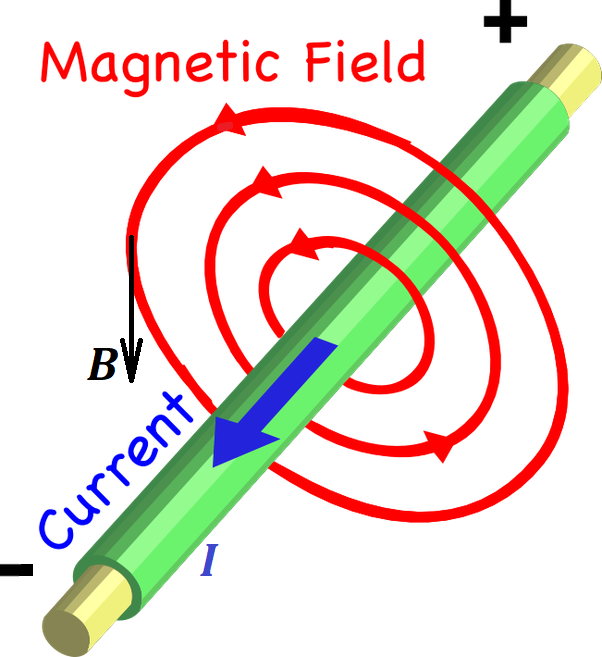The fact that electrons are moving signifies the existence of the electric field of some intensity, and the appearance of a magnetic field we attribute to properties of electric field.

The next logical step is that around a line of moving electrons, when the movement is not constant, but variable, there exists a variable magnetic field.
For example, if we have a wire loop and connect it to alternate current (AC) source of electricity that always changes the direction certain number of times per second, the magnetic field going through this wire loop also will change the direction of its magnetic field lines with each cycle of an electric current.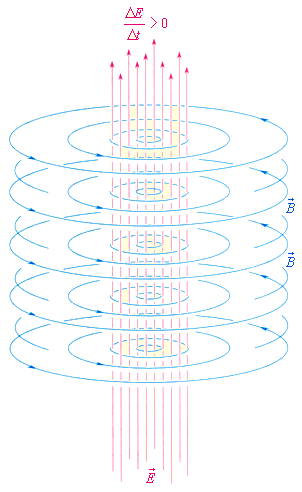The variability of the flow of electrons is caused by a variable electric field, and the appearance of a variable magnetic field we attribute to variability of an electric field.

The lecture "Straight Line Current" of this course (follow the menu items UNIZOR.COM - "Physics 4 Teens" - "Electromagnetism" - "Magnetism of Electric Current") explains this process in details for DC.
The lecture "Induction and AC" of this course (follow the menu items UNIZOR.COM - "Physics 4 Teens" - "Electromagnetism" - "Alternating Current Induction") explains this process in details for AC.

Our next experimental fact is the electromagnetic induction - a variable magnetic field around an electric wire causes appearance of a variable electric current in the wire that can only be caused by a variable electric field.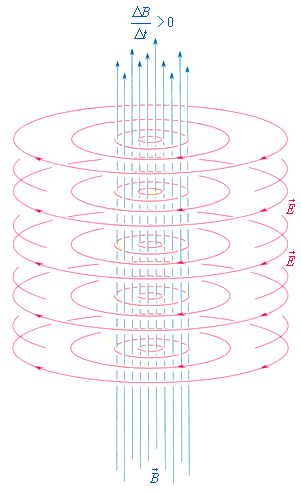So, we can conclude that a variable electric field causes the existence of a variable magnetic field, while a variable magnetic field causes the existence of a variable electric field.

Combining everything we mentioned above, we see that a variable electromagnetic field (a field where variable electric and magnetic forces exist) that consists of logically and physically chained together variable electric field and variable magnetic field, mutually causing each other, is self-sustained and capable of propagating through vacuum without existence of any wires as a medium.
This chain can schematically be represented as follows.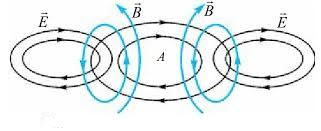Here a variable (oscillating) electric field causes the existence of a variable (oscillating) magnetic field in its neighborhood. That magnetic field causes the existence of another variable electric field further in space. This process repeats itself, thus facilitating the propagation of electromagnetic oscillations of an electromagnetic field.

Any field has certain forces acting on certain objects (or other fields).
The electromagnetic field at any point can be characterized by two forces - intensity vector of electric component of a field (E) and intensity vector of its magnetic component (B).

Here are a few facts about electromagnetic fields that require deeper immersion into their structure and are beyond the scope of this course.

1. Propagation of electromagnetic waves (called radiation in many cases) is perpendicular to both intensity vectors, electric and magnetic.
That makes these waves transverse.

2. Electric and magnetic intensity vectors are sinusoidal, perpendicular to each other and are in phase. Combining that property with their perpendicularity to a direction of propagation prompts the following graphical representation of electromagnetic waves.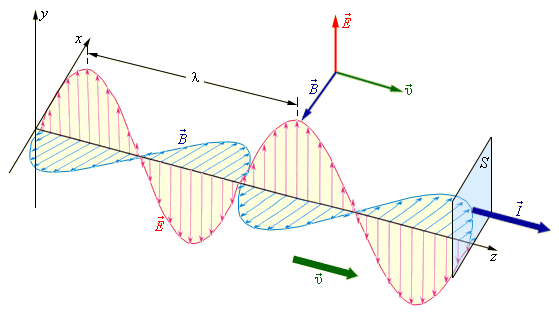(you can click the right mouse button and open this picture in another tab for better view)

3. Speed of propagation depends only on the properties of environment where the radiation is taking place.
In particular, the ability of electric field to permeate an environment (ε) and the ability of magnetic field to permeate an environment (μ) determine this speed.
The electric permittivity of vacuum is
ε0 ≅ 8.8542·10−12
.
The magnetic permeability of vacuum is
μ0 ≅ 1.2566·10−6
(H/m - Henry per meter)
.
Speed of electromagnetic waves c can be expressed in terms of electric permittivity and magnetic permeability as
c² = 1/(ε0·μ0)
According to the formula above and values of electric and magnetic constants, in vacuum the speed of electromagnetic waves is
c = 299,792,458 (m/sec)
which in many cases is rounded to 300,000 km/sec.

## Saturday, October 30, 2021

### Problems 1: UNIZOR.COM - Physics4Teens - Waves - Mechanical Oscillations

Notes to a video lecture on http://www.unizor.com

Problems 1

Problem A

Analyze the oscillations of a pendulum in a uniform gravitational field.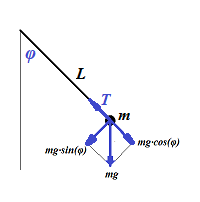Solution

The picture above defines a pendulum with a thread length L and a point-mass m hanging in a gravitational field that produces a constant gravity acceleration g.

Let angle φ be a deviation of the thread from a vertical. This angle is a function of time, so we will use notation φ(t) and will attempt to include it in some equation in order to find this function.

There are two forces acting on a point-mass m: gravity, directed vertically down and equal to m·g, and tension of a thread T.
The force of gravity can be represented as a sum of two forces: directed along a thread and equal to m·g·cos(φ(t)) and perpendicular to a thread equal to m·g·sin(φ(t)).

The tension force T balances the force m·g·cos(φ(t)), as both directed along a thread in opposite directions, thus canceling each other.
The force m·g·sin(φ(t)) is the source of pendulum moving back an force, as it is always directed towards a vertical position of equilibrium.

For the purposes of this analysis we assume that initially we have moved a pendulum from its initial position, so its thread makes an angle φ(0)=φ0 with a vertical and let it go without any push, so φ'(0)=0.

According to the Second Newton's Law, the force is equal to a product of the mass and its linear acceleration.
Therefore, the force F=m·g·sin(φ(t)) that always acts along a tangential to a trajectory of the point-mass towards its equilibrium point should be equal by absolute value to a product of mass m and linear acceleration along a circular trajectory on a thread of the length L, which is equal to L·φ"(t).

Also, this force F always acts towards the vertical. Choose a positive direction of the deviation φ from a vertical as counterclockwise. For positive angles φ, as on the picture above, the direction of the force F would be negative. For negative angles φ the direction of the force F would be positive.
Therefore, the signs of the force F and deviation from the vertical are opposite to each other. For small angles φ the sign of sin(φ) would be the same as the sign of φ.

It results in the following differential equation
−m·g·sin(φ(t)) = m·L·φ"(t)
or
φ"(t) + (g/L)·sin(φ(t)) = 0

Since mass m can be canceled out, our first very important conclusion is that pendulum oscillation does not depend on the mass of an object hanging at its end.

The differential equation above is quite complex and we will not attempt to solve it exactly. However, as many physicists do, we can approximate it under certain conditions.
The condition usually accepted is that the angle of deviation φ(t) is very small and, consequently, function sin (φ(t)) is almost identical to function φ(t).

That brings us to an equation
φ"(t) + (g/L)·φ(t) = 0
which is easy to solve, as it is a regular equation for harmonic oscillations.
The general solution to it, as explained in the previous lecture, is
φ(t) = C1·cos(ωt) + C2·sin(ωt)
where ω=√g/L.
Constants C1 and C2 are determined by initial conditions.

In case of initial conditions φ(0)=φ0 and φ'(0)=0 the values of these constants are:
C1 = φ0
C2 = 0

The solution of pendulum oscillations in this case is
φ(t) = φ0·cos(√g/L·t)
The angular frequency of these oscillations is
ω=√g/L.
The period of oscillations is
T = 2π/ω = 2π√L/g.
The amplitude is
A = φ0.

Just as a reminder, this analysis is applicable only to small oscillations of a pendulum around its equilibrium point, when an angle (in radians) and its sine are approximately equal to each other. Obviously, for any degree of precision the term "small" has its own meaning, the greater the precision - the smaller angle our analysis is applicable to.

Problem B

Analyze the oscillations of a ball of mass m rolling inside a semicircle of radius R in the uniform gravitational field with gravity acceleration g.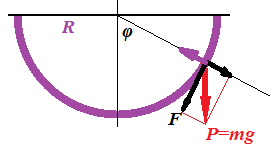Solution

The diagram above depicts the forces acting on a ball rolling inside a semicircle.

The weight P=m·g can be represented as a sum of tangential force F=P·sin(φ(t)) and normal to a circle force that is canceled by a reaction of a circle surface.
We should take into account that the direction of force F is always opposite in sign to a sign of angle φ(t). So, the correct expressions for force F is
F = −m·g·sin(φ(t))
According to Newton's Second Law, the force F can be equated to the product of mass and linear acceleration R·φ"(t) of the rolling ball.
F = m·R·φ"(t)
This can be expressed as a differential equation for an angle of position φ(t).
−m·g·sin(φ(t)) = m·R·φ"(t) or, canceling mass m and dividing by radius R,
φ"(t) + (g/R)·sin(φ(t)) = 0

As you see, the result is equivalent to the one we have obtained for a pendulum in the previous problem.

This differential equation is not easily solvable, so we will do exactly as in the previous problem - assume that an angle φ is so small that sin(φ(t)) can be approximated with φ(t), which leads us to a familiar differential equation for harmonic oscillations
φ"(t) + (g/R)·φ(t) = 0

General and specific solutions of this equation are exactly as in the case of a pendulum in Problem A above.